Introduction to Bayesian Analysis Procedures
 Summary Statistics

Let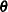be a-dimensional parameter vector of interest: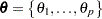. For each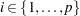, there areobservations: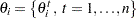.

### Mean

The posterior mean is calculated by using the following formula: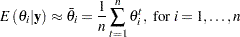### Standard Deviation

Sample standard deviation (expressed in variance term) is calculated by using the following formula: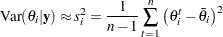### Standard Error of the Mean Estimate

Suppose you haveiid samples, the mean estimate is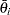, and the sample standard deviation is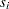. The standard error of the estimate is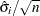. However, positive autocorrelation (see the section Autocorrelations for a definition) in the MCMC samples makes this an underestimate. To take account of the autocorrelation, the Bayesian procedures correct the standard error by using effective sample size (see the section Effective Sample Size).

Given an effective sample size of, the standard error foris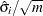. The procedures use the following formula (expressed in variance term):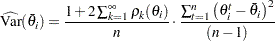The standard error of the mean is also known as the Monte Carlo standard error (MCSE). The MCSE provides a measurement of the accuracy of the posterior estimates, and small values do not necessarily indicate that you have recovered the true posterior mean.

### Percentiles

Sample percentiles are calculated using Definition 5 (see Chapter 4, The UNIVARIATE Procedure (Base SAS Procedures Guide: Statistical Procedures),).

### Correlation

Correlation between
and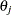is calculated as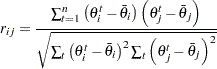### Covariance

Covarianceandis calculated as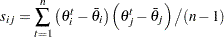### Equal-Tail Credible Interval

Let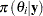denote the marginal posterior cumulative distribution function of. A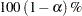Bayesian equal-tail credible interval foris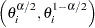, where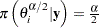, and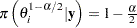. The interval is obtained using the empirical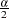th and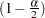th percentiles of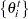.

### Highest Posterior Density (HPD) Interval

For a definition of an HPD interval, see the section Interval Estimation. The procedures use the Chen-Shao algorithm (Chen and Shao; 1999; Chen, Shao, and Ibrahim; 2000) to estimate an empirical HPD interval of:

1. Sort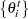to obtain the ordered values: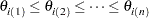2. Compute thecredible intervals: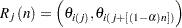for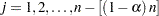.

3. TheHPD interval, denoted by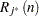, is the one with the smallest interval width among all credible intervals.

### Deviance Information Criterion (DIC)

The deviance information criterion (DIC) (Spiegelhalter et al.; 2002) is a model assessment tool, and it is a Bayesian alternative to Akaike’s information criterion (AIC) and the Bayesian information criterion (BIC, also known as the Schwarz criterion). The DIC uses the posterior densities, which means that it takes the prior information into account. The criterion can be applied to nonnested models and models that have non-iid data. Calculation of the DIC in MCMC is trivial—it does not require maximization over the parameter space, like the AIC and BIC. A smaller DIC indicates a better fit to the data set.

Lettingbe the parameters of the model, the deviance information formula is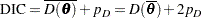where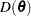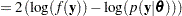: deviance

where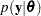: likelihood function with the normalizing constants.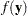: a standardizing term that is a function of the data alone. This term is constant with respect to the parameter and is irrelevant when you compare different models that have the same likelihood function. Since the term cancels out in DIC comparisons, its calculation is often omitted.

Note:You can think of the deviance as the difference in twice the log likelihood between the saturated,, and fitted,, models.
: posterior mean, approximated by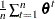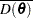: posterior mean of the deviance, approximated by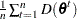. The expected deviation measures how well the model fits the data.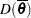: deviance evaluated at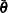, equal to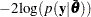. It is the deviance evaluated at your "best" posterior estimate.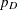: effective number of parameters. It is the difference between the measure of fit and the deviance at the estimates: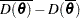. This term describes the complexity of the model, and it serves as a penalization term that corrects deviance’s propensity toward models with more parameters.Previous Page | Next Page | Top of Page